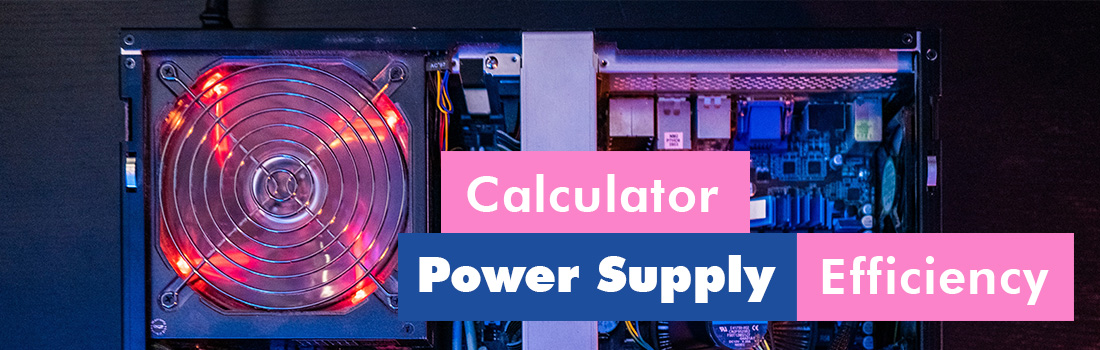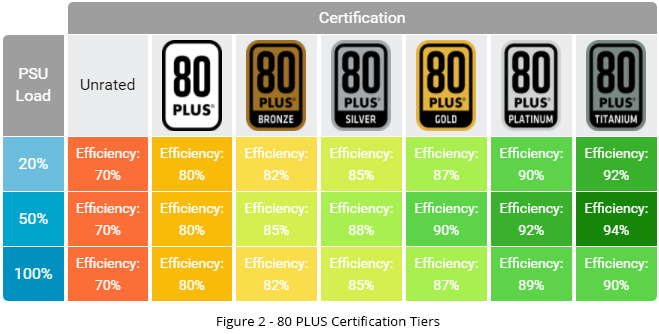# Psu Efficiency Calculator - How much money could you save?

Use our Psu Efficiency Calculator to see if it's worth investing in a better quality power supply. You can find out thanks to our psu efficiency savings calculator how much money you'll save per year if you buy a higher efficiency power supply.

## How does our Psu Efficiency Calculator work?

### ~ Step 1 ~

Enter your computer's consumption and how many hours your PC runs in a day into the calculator

### ~ Step 2 ~

Enter the energy price per kilowatt hour and select the efficiency of your power supply

### ~ Step 3 ~

The calculator will calculate how much you will save compared to the cheapest basic power supply.## What is Power supply?

An ATX computer power supply is a device used to process an AC power supply into the low voltage needed to power the individual components of a computer.

## 1. Power Usage

The power of a computer power supply is given in watts. Power reveals how many Watts the power supply is able to continuously supply to individual computer components. Depending on the amount of power, the use of a desktop computer can be characterized in a way. Computer power supplies with a wattage of around 300W - 400W are primarily intended for office work. As for gaming computers, their power ranges from above 400 W to values exceeding 1 000 W.

## How to find out the real consumption of my computer?

A wattmeter is a device used to find out the real power consumption of a computer. It is placed between the socket and the appliance. The wattmeter (power meter) is used to measure the electricity in real time. A wattmeter allows you to detect, measure and monitor your electricity consumption in a clear way. In addition, better wattmeters multiply the result directly by the price of energy.

## 2. What is Power supply Efficiency?

The efficiency of a power supply is the extent to which the power supply can efficiently use the current it draws from the grid. It is expressed as a percentage. The higher the efficiency, the less electricity is consumed for a given power output.Source of this image: Outervision

## Psu efficiency savings

It is good to think about the efficiency of the power supply when choosing a power supply for an office or home PC. By using a high efficiency model, you can save quite an interesting amount of money on electricity consumption in the order of tens of \$ per PC per year. High performance and efficiency have a positive impact on the long life of the power supply. A certified power supply with better efficiency heats up less and is less noisy.

## 3. Energy costs

The kilowatt hour (kWh) is one of the basic units by which we measure energy consumption. To give an idea, this value corresponds to the work of a machine with a power of one kilowatt for one hour.

• As a simple example, let's use a classic 10 W incandescent bulb. Let's say we want to find out what the bulb's power consumption was when it was on for 10 hours. Just multiply the two values together, and the result is the bulb's consumption for ten hours. We find that the consumption is 100 Wh.
• If the price for 1 kWh is \$0.2. Divide this amount by 1 000 to get a price of \$0.0002. We multiply this number by the resulting consumption of 100 Wh and find that ten hours of light cost us 0.02 cents.